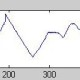# Spai

B'lore

I am a Ph.D student working on error control coding theory.
Top Questions

## How do we check if a polynomial is a perfect square?

asked Sep 4 '14 at 10:40

## The right way to motivate lattice theory in a combinatorics class

asked Apr 19 '13 at 15:58

## Are there infinitely many pairs of primes where each divides one more than the square of the other?

asked Jan 16 '15 at 20:20

## A trigonometric integral (guessed from a combinatorics formula)

asked May 20 '18 at 21:48

## How does one show that multiples of number does not have a zero in the decimal expansion?

asked May 9 '18 at 5:51

## A variant of an Eventown problem for modulo a prime number

asked Mar 14 '14 at 22:49